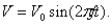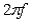Alternating-Current Circuits and Machines

# College Physics Reasoning and Relationships

Physics & Astronomy

## Quiz 22 :Alternating-Current Circuits and MachinesStudy FlashcardsLooking for Introductory Physics Homework Help?## Quiz 22 :Alternating-Current Circuits and Machines

Showing 1 - 20 of 64The output voltage of an AC generator is of the formA typical frequency in household circuits is 60 Hz. Using radians for angular measure in this equation, what is the factorin radians per second for this frequency?
Free
Multiple Choice

CIf the rotating coil used in a generator has its radius doubled, what change results in the amplitude of the voltage produced?
Free
Multiple Choice

BIn some countries the AC frequency is 50 Hz. What is the time interval for one cycle at this frequency?
Free
Multiple Choice

BWhat is the effective (rms) current value for an AC current with an amplitude of 20 A?
Multiple ChoiceIn an AC series circuit, the current in a pure resistor differs in phase with the applied voltage by what angle?
Multiple ChoiceThe rate of heat dissipation in an AC circuit with resistance, R, and effective current, Irms, is given by which of the following?
Multiple ChoiceAn AC voltage source, with a peak output of 200 V, is connected to a 25- resistor. What is the effective (or rms) current in the circuit?
Multiple ChoiceAn AC voltage source, with a peak output of 200 V, is connected to a 25- resistor. What is the rate of energy dissipated due to heat in the resistor?
Multiple ChoiceThe rms current is equal to the direct current that:
Multiple ChoiceAn AC voltage source, with a peak output of 120 V, results in dissipation of energy in a resistor at rate of 50.0 W. What is the value of the resistance?
Multiple ChoiceThe peak voltage of an AC source is 400 V. What is the rms voltage?
Multiple ChoiceIn the typical household AC voltage of 120 V, what is the peak voltage?
Multiple ChoiceThe frequency in an AC series circuit is halved. By what factor does this change the capacitive reactance?
Multiple ChoiceIn an AC series circuit the capacitive reactance is 253 and frequency is 100 Hz. What is the capacitance?
Multiple ChoiceA 17.0-F capacitor is connected to an AC source with an rms voltage of 120 V and a frequency of 60.0 Hz. What is the rms current in the capacitor?
Multiple ChoiceWhen a 50-F capacitor is attached to an AC source, its capacitive reactance is 60 . If instead a 200-F capacitor is attached to the same source, what will be its capacitive reactance?
Multiple ChoiceIn a capacitor in an AC circuit, the voltage:
Multiple ChoiceA 400-resistor and a capacitor are connected in parallel across a 300-Hz source. If the amplitude of the current in the resistor is equal to that in the capacitor, what is the value of the capacitance?
Multiple Choice